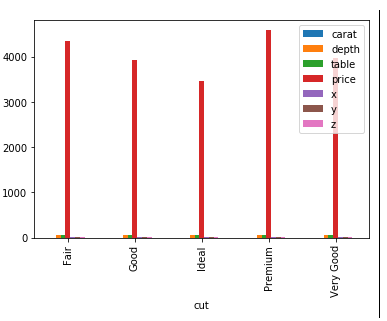﻿ Pandas Practice Set-1: Create a side-by-side bar plot of the diamonds DataFrame - w3resource# Pandas Practice Set-1: Create a side-by-side bar plot of the diamonds DataFrame

## Pandas Practice Set-1: Exercise-30 with Solution

Write a Pandas program to create a side-by-side bar plot of the diamonds DataFrame.

Sample Solution:

Python Code:

``````import pandas as pd
print("Original Dataframe:")
print("\nSide-by-side bar plot of the diamonds DataFrame:")
diamonds.groupby('cut').mean().plot(kind='bar')
``````

Sample Output:

```Original Dataframe:
carat      cut color clarity  depth  table  price     x     y     z
0   0.23    Ideal     E     SI2   61.5   55.0    326  3.95  3.98  2.43
1   0.21  Premium     E     SI1   59.8   61.0    326  3.89  3.84  2.31
2   0.23     Good     E     VS1   56.9   65.0    327  4.05  4.07  2.31
3   0.29  Premium     I     VS2   62.4   58.0    334  4.20  4.23  2.63
4   0.31     Good     J     SI2   63.3   58.0    335  4.34  4.35  2.75

Side-by-side bar plot of the diamonds DataFrame:```

Python Code Editor:

Have another way to solve this solution? Contribute your code (and comments) through Disqus.

What is the difficulty level of this exercise?

﻿

## Python: Tips of the Day

How to sort a Python dict by value

Example:

```x1 = {'a': 5, 'b': 7, 'c': 9, 'd': 1}

sorted(x1.items(), key=lambda x: x)
[('d', 1), ('c', 9), ('b', 7), ('a', 5)]

# Or:

import operator
print(sorted(x1.items(), key=operator.itemgetter(1)))
```

Output:

```[('d', 1), ('a', 5), ('b', 7), ('c', 9)]
```